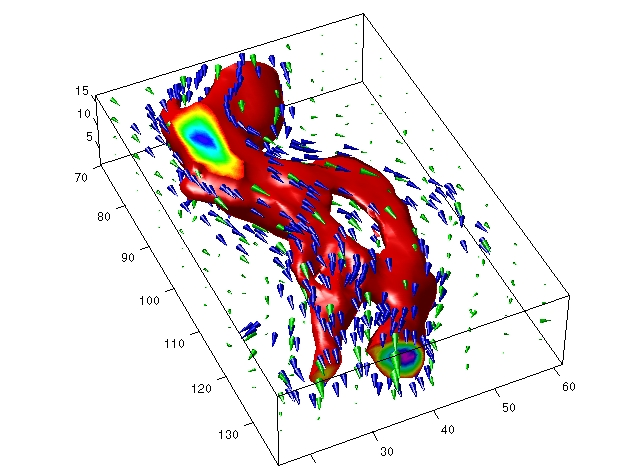3-D VisualizationVector Field Displayed with Cone Plots

This example plots the velocity vector cones for the `wind` data. The graph produced employs a number of visualization techniques:

• An isosurface is used to provide visual context for the cone plots and to provide means to select a specific data value for a set of cones.
• Lighting enables the shape of the isosurface to be clearly visible.
• The use of perspective projection, camera positioning, and view angle adjustments composes the final view.

1. Create an Isosurface

Displaying an isosurface within the rectangular space of the data provides a visual context for the cone plot. Creating the isosurface requires a number of steps:

• Calculate the magnitude of the vector field, which represents the speed of the wind.
• Use `isosurface` and `patch` to draw an isosurface illustrating where in the rectangular space the wind speed is equal to a particular value. Regions inside the isosurface have higher wind speeds, regions outside the isosurface have lower wind speeds.
• Use `isonormals` to compute vertex normals of the isosurface from the volume data rather than calculate the normals from the triangles used to render the isosurface. These normals generally produce more accurate results.
• Set visual properties of the isosurface, making it red and without drawing edges (`FaceColor`, `EdgeColor`).
• ```load wind
wind_speed = sqrt(u.^2 + v.^2 + w.^2);
hiso = patch(isosurface(x,y,z,wind_speed,40));
isonormals(x,y,z,wind_speed,hiso)
set(hiso,'FaceColor','red','EdgeColor','none');
```

2. Add Isocaps to the Isosurface

Isocaps are similar to slice planes in that they show a cross section of the volume. They are designed to be the end caps of isosurfaces. Using interpolated face color on an isocap causes a mapping of data value to color in the current colormap. To create isocaps for the isosurface, define them at the same isovalue (`isocaps`, `patch`, `colormap`).

• ```hcap = patch(isocaps(x,y,z,wind_speed,40),...
'FaceColor','interp',...
'EdgeColor','none');
colormap hsv
```

3. Create First Set of Cones

• Use `daspect` to set the data aspect ratio of the axes before calling `coneplot` so MATLAB can determine the proper size of the cones.
• Determine the points at which to place cones by calculating another isosurface that has a smaller isovalue (so the cones are displayed outside the first isosurface) and use `reducepatch` to reduce the number of faces and vertices (so there are not too many cones on the graph).
• Draw the cones and set the face color to blue and the edge color to none.
• ```daspect([1,1,1]);
[f verts] = reducepatch(isosurface(x,y,z,wind_speed,30),0.07);
h1 = coneplot(x,y,z,u,v,w,verts(:,1),verts(:,2),verts(:,3),3);
set(h1,'FaceColor','blue','EdgeColor','none');
```

4. Create Second Set of Cones

• Create a second set of points at values that span the data range (`linspace`, `meshgrid`).
• Draw a second set of cones and set the face color to green and the edge color to none.
• ```xrange = linspace(min(x(:)),max(x(:)),10);
yrange = linspace(min(y(:)),max(y(:)),10);
zrange = 3:4:15;
[cx,cy,cz] = meshgrid(xrange,yrange,zrange);
h2 = coneplot(x,y,z,u,v,w,cx,cy,cz,2);
set(h2,'FaceColor','green','EdgeColor','none');
```

5. Define the View

• Use the `axis` command to set the axis limits equal to the minimum and maximum values of the data and enclose the graph in a box to improve the sense of a volume (`box`).
• Set the projection type to perspective to create a more natural view of the volume. Set the viewpoint and zoom in to make the scene larger (`camproj`, `camzoom`, `view`).
• ```axis tight
box on
camproj perspective
camzoom(1.25)
view(65,45)
```

6. Add Lighting

Add a light source and use Phong lighting for the smoothest lighting of the isosurface (Phong lighting requires the Z-buffer renderer). Increase the strength of the background lighting on the isocaps to make them brighter (`camlight`, `lighting`, `AmbientStrength`).

• ```camlight(-45,45)
set(gcf,'Renderer','zbuffer');
lighting phong
set(hcap,'AmbientStrength',.6)```Creating Stream Particle Animations

© 1994-2005 The MathWorks, Inc.